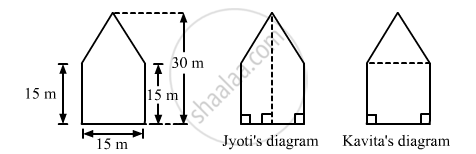Share

# There is a Pentagonal Shaped Park as Shown in Fig. 20.50. Jyoti and Kavita Divided It in Two Different Ways. - Mathematics

Course
ConceptArea of Trapezium

#### Question

There is a pentagonal shaped park as shown in Fig. 20.50. Jyoti and Kavita divided it in two different ways.Find the area of this park using both ways. Can you suggest some another way of finding its areas?

#### Solution

A pentagonal park is given below:Jyoti and Kavita divided it in two different ways.
(i) Jyoti divided is into two trapeziums as shown below:
It is clear that the park is divided in two equal trapeziums whose parallel sides are 30 m and 15 m.
And, the distance between the two parallel lines $:\frac{15}{2}=7.5 m$
∴ Area of the park=2 x (Area of a trapazium
$= 2 \times [\frac{1}{2} \times (30 + 15) \times (7 . 5)]$
${=337.5 m}^2$
$(ii)$
Kavita divided the park into a rectangle and a triangle, as shown in the figure.
Here, the height of the triangle = 30-15=15 m
∴ Area of the park=(Area of square with sides 15 cm)+(Area of triangle with base 15 m and altitude 15 m)
$=(15\times15)+(\frac{1}{2}\times15\times15)$
$= 225 + 112 . 5$
${=337.5 m}^2$

Is there an error in this question or solution?

#### APPEARS IN

RD Sharma Solution for Mathematics for Class 8 by R D Sharma (2019-2020 Session) (2017 to Current)
Chapter 20: Mensuration - I (Area of a Trapezium and a Polygon)
Ex. 20.3 | Q: 3 | Page no. 28

#### Video TutorialsVIEW ALL 

Solution There is a Pentagonal Shaped Park as Shown in Fig. 20.50. Jyoti and Kavita Divided It in Two Different Ways. Concept: Area of Trapezium.
S